### Home > CALC > Chapter 2 > Lesson 2.2.1 > Problem2-51

2-51.
1. Sketch the graph of the two functions below. Compare the equations and their graphs. Then write a complete set of approach statements for each. Homework Help ✎

1.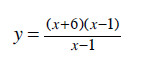2.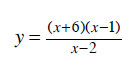3. Explain why one graph has a hole while the other has a vertical asymptote.

4. Find the end behavior of each graph.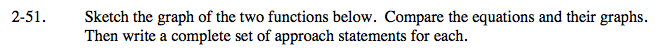For parts (a) and (b).
One of the functions has a hole and the other has an asymptote.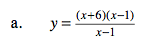Refer to Lesson 1.2.3, problems 1-44 & 1-45.

A complete set of approach statements include:

x → −∞
x → (hole)
x → (vertical asymptote)
x → ∞
x → (hole)+
x → (vertical asymptote)+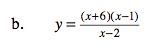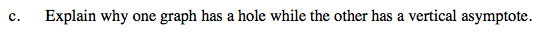How does algebra help determine the location of holes and vertical asymptotes?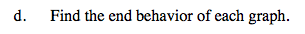Horizontal asymptotes, if they exist, are the same as end behavior. If there is no horizontal asymptote, find end behavior with polynomial division (ignore the remainder).